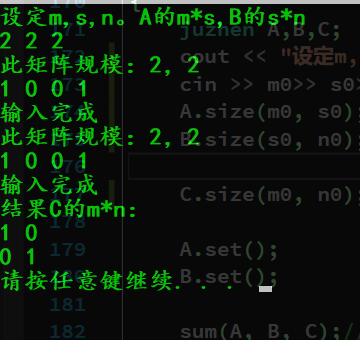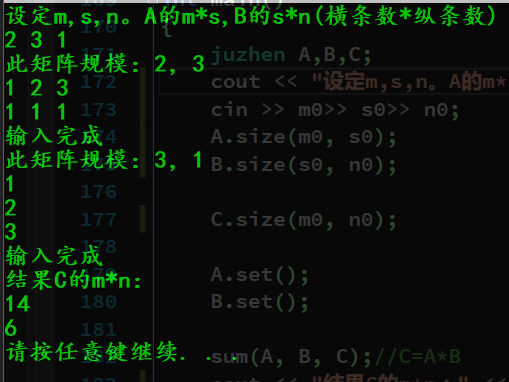• ## C++矩阵相乘

万次阅读 2018-06-09 15:36:13
//矩阵相乘     for  ( int  i = 0; i < rowA; ++i)   {    for  ( int  j = 0; j < colB; ++j)   {    for  ( int  k = 0; k < colA; ++k)   {   res...
转自：

https://blog.csdn.net/cxc19890214/article/details/45363267/

https://blog.csdn.net/yang20141109/article/details/51024373

方法一：数组实现：

#include<iostream>
using namespace std;
#define SIZE_M 2
#define SIZE_N 3
#define SIZE_S 4

int main(){

int matrix_a[SIZE_M] [SIZE_N]={{1,2,3},{4,5,6}};//a矩阵2X3
int matrix_b[SIZE_N] [SIZE_S]={{1,2,3,4},{5,6,7,8},{1,2,3,4}};//b矩阵3X4
int matrix_result[SIZE_M][SIZE_S];//结果矩阵2X4

for(int m=0;m<SIZE_M;m++){
for(int s=0;s<SIZE_S;s++){
matrix_result[m][s]=0;//变量使用前记得初始化,否则结果具有不确定性
for(int n=0;n<SIZE_N;n++){
matrix_result[m][s]+=matrix_a[m][n]*matrix_b[n][s];
}
}
}
for(int m=0;m<SIZE_M;m++){
for(int s=0;s<SIZE_S;s++){
cout<<matrix_result[m][s]<<"\t";
}
cout<<endl;
}

return 0;

}
方法二：vector实现

#include<iostream>
#include<vector>
using namespace std;

vector<vector<int>> matrix_multiply(vector<vector<int>> arrA, vector<vector<int>> arrB)
{
//矩阵arrA的行数
int rowA = arrA.size();
//矩阵arrA的列数
int colA = arrA.size();
//矩阵arrB的行数
int rowB = arrB.size();
//矩阵arrB的列数
int colB = arrB.size();
//相乘后的结果矩阵
vector<vector<int>>  res;
if (colA != rowB)//如果矩阵arrA的列数不等于矩阵arrB的行数。则返回空
{
return res;
}
else
{
//设置结果矩阵的大小，初始化为为0
res.resize(rowA);
for (int i = 0; i < rowA; ++i)
{
res[i].resize(colB);
}

//矩阵相乘
for (int i = 0; i < rowA; ++i)
{
for (int j = 0; j < colB; ++j)
{
for (int k = 0; k < colA; ++k)
{
res[i][j] += arrA[i][k] * arrB[k][j];
}
}
}
}
return res;
}

int main(void)
{
vector<vector<int>> arrA = { { 2, 1 }, { 4, 3 } };
vector<vector<int>> arrB = { { 1, 2 }, { 1, 0 } };
//vector<vector<int>> arrA = { { 1, 2, 3 }, { 4, 5, 6 } };
//vector<vector<int>> arrB = { { 1, 4 }, { 2, 5 }, { 3, 6 } };
vector<vector<int>> res = matrix_multiply(arrA, arrB);
system("pause");
return 0;
}

展开全文• 矩阵相乘最重要的方法是一般矩阵乘积。它只有在第一个矩阵的列（column）和第二个矩阵的行数（row）相同时才有意义 。一般单指矩阵乘积时，指的便是一般矩阵乘积。一个m×n的矩阵就是m×n个数排成m行n列的一个数阵。...
矩阵相乘最重要的方法是一般矩阵乘积。它只有在第一个矩阵的列（column）和第二个矩阵的行数（row）相同时才有意义 。一般单指矩阵乘积时，指的便是一般矩阵乘积。一个m×n的矩阵就是m×n个数排成m行n列的一个数阵。由于它把许多数据紧凑的集中到了一起，所以有时候可以简便地表示一些复杂的模型。
运算如下所示：
我们可以在2个矩阵上执行加，减，乘和除运算。从用户输入一行数字和列号，组成第一个矩阵元素和第二个矩阵元素。然后，对用户输入的矩阵执行乘法。
1.思路
首先，由于输入的矩阵维数是随机的，因此，我们要设计程序，手动把行和列算出来，这样方便后续乘法运算。并且把输入的数字提取出来，放入一个float型数组中，这样我们就完成了读入工作，之后就是利用乘法公式进行运算，并把结果放入一个二维数组中，最后把结果输出来就行了。
2.数据读入
这里是容易出现问题的地方，最初的想法是用cin.getline()把整个输入都读进一个char型字符序列中，然后再用特定位置的数做乘法。后来发现有两个问题，第一，数字读入一个char字符序列中就变成了ASCII码，这个还比较好解决，用每个位置的数减去‘ 0‘就行了。第二个问题是硬伤，就是把一个数字放到一个char型的序列中，他会把连在一起的数字给拆开，比如说我想输入123，他不会把123放到一个格里，而是1放到一个格，2放入另一个格，3再放一个格。所以不能放到char[]中。于是想到把输入放到float数组里， 但是这样就有一个新问题，就是如何把符号摘出去。如果直接用cin，那么碰到符号它并不会跳过，而是也会录入，这是不行的，但是对于这个问题，我们知道输入的格式都是类似于：123，1，2；1，2，3这样的，规律就是一个数字一个符号，我们可以用赋值的方式来跳过，和；的录入。具体来说就是先用一个cin，把第一个数字录入，然后用c=getchar（）的方式来跳过逗号的录入。然后再cin，再c=getchar，最终当c=getchar（）等于回车，也就是\n时停止。由于c=getchar（）是判断条件，所以我们这个循环要从逗号开始，也就是先录入一个数字，再进行循环。
  在录入的过程中，我们就可以直接把行数和列数读取出来：行数就是；（分号）的个数加一，列数就是总共的数字个数除以行数。



cout << "please enter matrixA:"<<endl;
cin >> A[i];
while ((c=getchar()) != '\n')
{
cin >> A[++i];
if (c == ';')m++;
}
m++;
i++;
n = i/ m;
cout << "please enter matrixB:"<<endl;
cin >> B[j];
while ((c = getchar()) != '\n')
{
cin >> B[++j];
if (c == ';')
k++;
}
k++;
j++;
p = j / k;

  拿第一段，矩阵A的录入来说，m是行数，由于m作为；的计数器，因此行数要在分号个数的基础上加一，即m++，而由于A[ ]这个数组是从A开始的，因此A[i]表示有i+1个数，所以i++。这样就实现了矩阵A，B的录入，虽然录进去的是一个一维的数组，但也不妨碍后续的矩阵乘法计算。

3.矩阵相乘

在矩阵乘法第一矩阵中，一个行元素乘以第二矩阵所有列元素。让我们通过下面的代码理解3 * 3和3 * 3矩阵的矩阵乘法：

下面来看看看C++中的矩阵乘法程序。
#include <iostream>
using namespace std;
int main()
{
int a,b,mul,r,c,i,j,k;
cout<<"enter the number of row=";
cin>>r;
cout<<"enter the number of column=";
cin>>c;
cout<<"enter the first matrix element=\n";
for(i=0;i<r;i++)
{
for(j=0;j<c;j++)
{
cin>>a[i][j];
}
}
cout<<"enter the second matrix element=\n";
for(i=0;i<r;i++)
{
for(j=0;j<c;j++)
{
cin>>b[i][j];
}
}
cout<<"multiply of the matrix=\n";
for(i=0;i<r;i++)
{
for(j=0;j<c;j++)
{
mul[i][j]=0;
for(k=0;k<c;k++)
{
mul[i][j]+=a[i][k]*b[k][j];
}
}
}
//for printing result
for(i=0;i<r;i++)
{
for(j=0;j<c;j++)
{
cout<<mul[i][j]<<" ";
}
cout<<"\n";
}
return 0;
}

执行上面代码得到以下结果
enter the number of row=3
enter the number of column=3
enter the first matrix element=
1 2 3
1 2 3
1 2 3
enter the second matrix element=
1 1 1
2 1 2
3 2 1
multiply of the matrix=
14 9 8
14 9 8
14 9 8


展开全文c语言
• #include<bits/stdc++.h> using namespace std; typedef long long ll; typedef vector<double> vec; typedef vector<vec> mat; const int INF=0x3f3f3f3f; ...mat mul(mat...
#include<bits/stdc++.h>
using namespace std;

typedef long long ll;
typedef vector<double> vec;
typedef vector<vec> mat;

const int INF=0x3f3f3f3f;
const int MAX_N=100;

mat mul(mat &A,mat &B){
mat C(A.size(),vec(B.size()));
for(int i=0;i<A.size();i++){
for(int j=0;j<B.size();j++){
for(int k=0;k<A.size();k++){
C[i][j]+=A[i][k]*B[k][j];
}
}
}
return C;
}

mat qpow(mat A,ll n){
mat B(A.size(),vec(A.size()));
for(int i=0;i<B.size();i++)B[i][i]=1;
while(n>0){
if(n&1)B=mul(B,A);
A=mul(A,A);
n>>=1;
}
return B;
}

void show(mat &A){
for(int i=0;i<A.size();i++){
for(int j=0;j<A.size();j++){
cout<<A[i][j]<<" ";
}
cout<<endl;
}
}




展开全文• 复习数学1的线性代数，矩阵相乘这块有点晕，想编个C++对象化的矩阵相乘小程序…… 相乘部分 void sum(juzhen a, juzhen b, juzhen &c) { int s=0; for (int i = 1; i (); i++)//A矩阵的M for (int j = 1; j ()...
复习数学1的线性代数，矩阵相乘这块有点晕，想编个C++对象化的矩阵相乘小程序……

相乘部分

void sum(juzhen a, juzhen b, juzhen &c)
{
int s=0;
for (int i = 1; i <= a.m1(); i++)//A矩阵的M
for (int j = 1; j <= b.n1(); j++)//B矩阵的S
{
for (k0 = 1; k0 <= a.n1(); k0++)//a.n1也就是b.m1(a的n，b的n)【行向量*列向量】
{
s += a.read(i,k0)*b.read(k0,j);
}
c.write(i, j, s);
s = 0;
}
}

公式：代码中的头两个for循环就是i，j的。公式中的k从1到p求和就是里面的k0的for循环。
容易出现误解的就是公式中只是表示第“[i][j]”元素，而不是整个矩阵，整个矩阵的结果需要外面的两个for循环。
本质：这就是个p维向量（高中就记2维）的两向量相乘公式而已【结果为数，是新矩阵的一个元素】

可运行代码：
#include<iostream>
#include <string>
using namespace std;

class juzhen
{
private:
int m,n;//长宽
int num = {0};
string name;

public:
void size(int a,int b)
{	m = a;
n = b; }
void set()
{
cout << "此矩阵规模：" << this->m <<"，"<< this->n << endl;//=====？
for (int i = 1; i <= this->m; i++)
for (int j = 1; j <= this->n; j++)
{
cin >> this->num[i][j];
}
cout << "输入完成"<< endl;
}
void display()
{
for (int i = 1; i <= this->m; i++)//===i为行号（第几行），j为列号
for (int j = 1; j <= this->n; j++)
{
cout << this->num[i][j] << " ";
if (j == this->n) cout << endl;//先输出再换行
}
}
int read(int a, int b) { return num[a][b]; }//调用此函数，得[m][n]元素的值
void write(int a, int b,int count) {  num[a][b]=count; }//第三个参数的值，传递给[a][b]元素
int m1() { return m; }//调用得到矩阵的m
int n1() { return n; }//调用得到矩阵的n
};

int m0, n0, s0, k0;//矩阵规模（容易搞混的东西）

void sum(juzhen a, juzhen b, juzhen &c)//矩阵相乘公式所在。。。【要改实参值的要用&引用】
{
for (int i = 1; i <= a.m1(); i++)//A矩阵的M
for (int j = 1; j <= b.n1(); j++)//B矩阵的S
{

int s = 0;
for (k0 = 1; k0 <= a.n1(); k0++)//a.n1也就是b.m1(a的n，b的n)【行向量*列向量】
{
s += a.read(i,k0)*b.read(k0,j);//因为用了C++，所以没那么直观，就是a[i][k]*b[k][j]，套个for循环求累加和（就是高中时向量的点乘）
}
c.write(i, j, s);
}
}
int main()
{
juzhen A,B,C;
cout << "设定m,s,n。A的m*s,B的s*n(横条数*纵条数)"<<endl;
cin >> m0>> s0>> n0;
A.size(m0, s0);
B.size(s0, n0);

C.size(m0, n0);

A.set();
B.set();

sum(A, B, C);//C=A*B
cout << "结果C的m*n：" << endl;
C.display();

return 0;
}


样例输入及输出：
1 0          1 0            1 0
0 1      *   0 1      =   0 1懒得打了……就如图所示两个矩阵PS：函数中形参引用真好用，过去一直不知道，省得用指针了。（不然改不了C矩阵的实际元素值）

void sum(juzhen a, juzhen b, juzhen &c)//矩阵相乘公式所在。。。【要改实参值的要用&引用】


展开全文• /*--------------------------计算NBB矩阵----------------------------*/ /*----------------------计算W矩阵-------------------------------*/ NBB = 0; for (int i = 0; i < 3; i++) for ...
• /*--------------------------计算NBB矩阵----------------------------*/ /*----------------------计算W矩阵-------------------------------*/ NBB = 0; for (int i = 0; i < 3; i++) for (int j = ...
• } /*--------------------------计算NBB矩阵----------------------------*/ /*----------------------计算W矩阵-------------------------------*/ NBB = 0; for (int i = 0; i < 3; i++) for (int j = ...
• 相信很多人在二维数组相乘的时候发现了值出错的问题 其实是很小的问题。原因在于我们在使用+=的时候第一次循环的时候数组的值是空造成+=的时候第一个数据是指针造成程序错误；
• C++类的方法来实现两个矩阵相乘得到一个新的矩阵
• 参考自：... #include <iostream> #include <amp.h>...using namespace concurrency;...// 常规矩阵相乘计算 void MultiplyWithOutAMP() { int aM...
• 矩阵相乘multiply 求矩阵的逆矩阵inverse 有了这三个方法，足以进行机器人正逆运动学分析了。 直接上代码： #include&amp;amp;amp;lt;iostream&amp;amp;amp;gt; #include&amp;amp;amp;lt;stdlib.h&...
• 矩阵相乘c++实现，可以参考借鉴，两个矩阵相乘
• 很惭愧地说，之前矩阵相乘一直在调函数，从来没有自己实现过，自己是非计算机科班出身，感觉基础确实一般。所以今天试着写了个多线程矩阵乘法作为练习，看看计算效率如何，如有问题欢迎交流指正。这里矩阵用的是 ...
• 编程求两个矩阵相乘的结果。输入第一行是整数m,n,表示第一个矩阵式m行n列的；然后是一个m * n的矩阵。 再下一行的输入时整数p,q，表示下一个矩阵p行,q列的（n=p）;然后就是一个p行q列的矩阵。 要求输出两个矩阵相乘...
• 矩阵相乘 今天又是闲来无事的一天，在网上又看到了这道矩阵相乘的题。不知道为什么，可能是高数学太久忘了，就想把这道题写下来。记录一下高数知识。 题目描述 给定一个N阶矩阵A，输出A的M次幂（M是非负整数） ...
• 自由定义矩阵相乘 #include using namespace std; void main() { int a={0},b={0},c={0}; int i,j,m,n,k,x,y; cout<<“please input the matrex_a that is mn and matrex_b that is ...
•  C/C++实现矩阵相乘(一)从文件中读取矩阵，相乘并得到结果。下面的代码同样实现矩阵相乘，不过完全在控制 台进行，比较简单 #include #include int main(){ int ROW1=0, COL1=0, ROW2=0, COL2=0,NUM=0,RESNUM=0; ...malloc
• void matrixMulti( int **a, int **b,int **c,int n1,int m,int n2) {  for (int i=0; i  {  for (int j=0; j  {delete null c
• //矩阵相乘 for (int i = 0; i ; ++i) { for (int j = 0; j ; ++j) { for (int k = 0; k ; ++k) { res[i][j] += arrA[i][k] * arrB[k][j]; } } } } return res; } int main...
• 编写函数，使用指针传递和指针运算，实现两个矩阵的乘积。 编写主程序，输入两个矩阵，调用函数计算它们的乘积，在主函数中输出计算结果。每个矩阵的元素总个数不超过100，但行数、列数不定。矩阵元素为整数。 输入...指针
• 原文链接：C++实现矩阵相乘 #include<iostream> using namespace std; #define SIZE_M 2 #define SIZE_N 3 #define SIZE_S 4 int main(){ int matrix_a[SIZE_M] [SIZE_N]={{1,2,3},{4,5,6}};//a矩阵2X3 ...
• Strassen 算法 C++ 实现 任意矩阵相乘 用Command line 输入编制好的两个矩阵，输出相乘的结果矩阵。如果需要手动输入，删除程序里的相关语句，添加输入命令即可。...

# c++矩阵相乘c++ 订阅